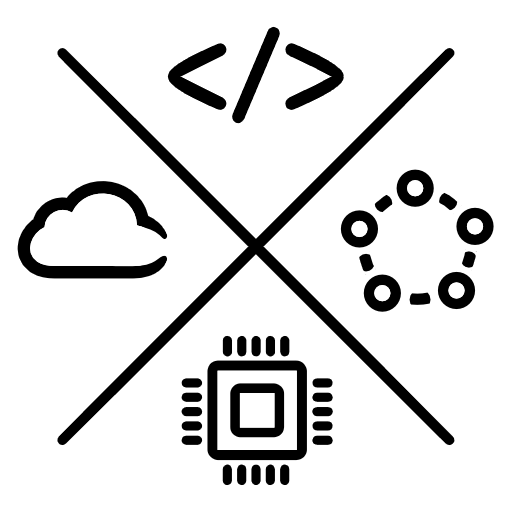Roman Parykin

Software development, distributed systems, & natural language processing

# Scaling effects in Scala

Feb 10, 2019

42. A value. Different from 37 but both are numbers.

Effect is to type what type is to value.

🄰s type allows to abstract over value, effect allows to abstract over type.

``````42: Int
"text": String
``````
``````def opt[A](value: A): Option[A]
opt(42): Option[Int]
opt("text"): Option[String]
``````

There are two types of effect scaling—horizontal ⇄ and vertical ⇅.

## Horizontal Scaling

The simplest scaling approach that replaces an effect with another one.

Built-in effects: `Option[A] → Future[A]`.

Third-party effects: `Future[A] → Task[A]`. https://monix.io

``````import scala.concurrent.Future
import monix.execution.Scheduler.Implicits.global

val future = Future(42)

``````

Horizontal scaling is bidirectional if types are isomorphic and unidirectional otherwise.

## Vertical Scaling

⼐ With ability to abstract over types the next step is to abstract over effects.

Algebra is to effect what effect is to type.

One way to abstract over effects is the tagless final encoding.

``````import java.util.UUID

final case class Snapshot(tenantId: UUID, id: UUID)

trait SnapshotRepo[F[_]] {
def current(tenantId: UUID): F[Option[Snapshot]]
}
``````

``````import cats.~>
import cats.free.Free

sealed trait ObjectStoreAlgebra[A]
final case class Put(path: String, bytes: Array[Byte]) extends ObjectStoreAlgebra[Unit]

object ObjectStore {
type ObjectStore[A] = Free[ObjectStoreAlgebra, A]

def put(path: String, bytes: Array[Byte]): ObjectStore[Unit] =
Free.liftF[ObjectStoreAlgebra, Unit](Put(path, bytes))
}

def compiler[F[_]]: ObjectStoreAlgebra ~> F = ???
``````

⇄ Algebras can be scaled horizontally by replacing one approach with another.

Extensible effects are to algebras what algebras are to effects. https://atnos-org.github.io/eff

``````import cats.Monad
import cats.data.Writer
import org.atnos.eff._
import org.atnos.eff.all._

sealed trait Event
final case class ObjectStored(path: String) extends Event

type _effect[R] = F |= R                    // Expect to deal with F effect
type _writer[R] = Writer[String, ?] |= R    // And the Cats' Writer for logging

def process[R: _option: _effect: _writer](  // Expect to see some Options
tenantId:     UUID,
snapshotRepo: SnapshotRepo[F],            // Pass tagless final interpreter
compiler:     ObjectStoreAlgebra ~> F     // Pass free monad compiler
)(implicit M: Monad[F]): Eff[R, Event] = for {
_ ← tell("Starting processing")                                 // Cats data type
maybeSnapshot ← Eff.send(snapshotRepo.current(tenantId))        // Tagless final algebra effect
snapshot ← fromOption(maybeSnapshot)                            // Built-in Option type
path = s"\${tenantId}/\${snapshot.id}"                            // A value
} yield ObjectStored(path)
}
``````

⇅ Vertical scaling implies both scaling up and scaling down.

☰ Different effects along with algebra interpreters are computed in one place. https://typelevel.org/cats/datatypes/writer.html

↓ The API is scaled down being encoded as tagless final `trait PayloadAlgebra[F[_]]`.

↓ Further downscaling is achieved by running the computation.

``````type Stack = Fx.fx3[Option, Task, Writer[String, ?]]

.runOption
.runWriterUnsafe[String](println)
.runAsync
``````

⧇ Using the abstract interface, the computation results in nested effects.

⟷ The effect can be scaled horizontally as at the beginning.

```flowchart BT
subgraph Effects
direction LR
option["Option"]
cats["Cats Writer"]
future["Future"]
option -.- cats -.- future <-..-> task
end

subgraph Algebras
direction LR
tf[Tagless final]